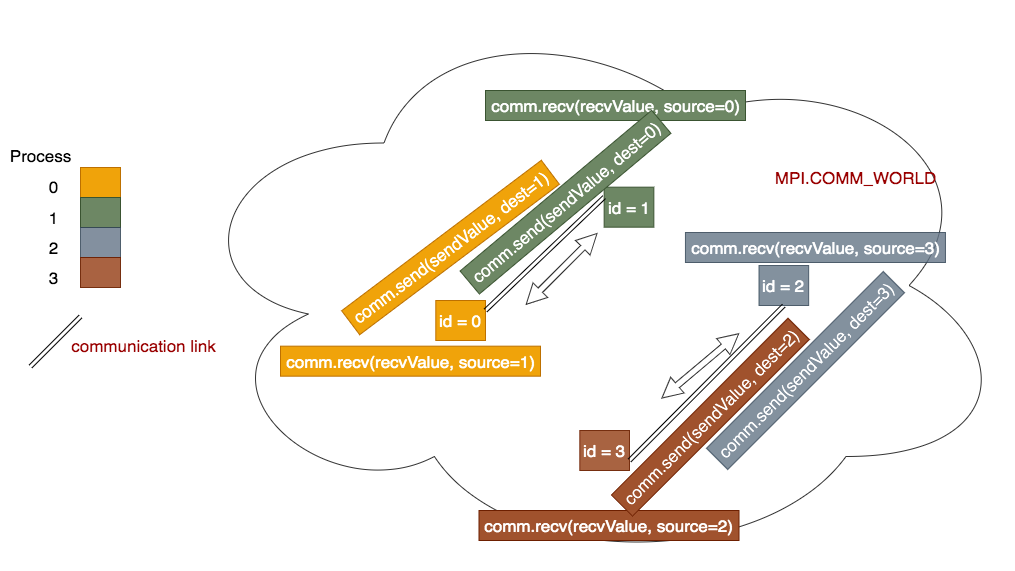A common source of errors in programs that use point-to-point communication is called deadlock. In this section, we define what deadlock is, and how to fix it.

In the point-to-point communication programs we have looked at so far, we have one process that is sending messages, and another process that is receiving it. Consider the case where two processes exchange data:When two or more procesess exchange data, each process performs both a send and a receive. The idea is that process 0 will send data to process 1, who will receive it from process 0. Process 1 will also send some data to process 0, who will receive it from process 1. Similarly, processes 2 and 3 will exchange messages: process 2 will send data to process 3, who will receive it from process 2. Process 3 will also send some data to process 2, who will receive it from process 3.

Deadlock occurs when every process is forced to wait on an action of another process to continue executing. Since all the processes are waiting (or blocked) on another waiting process, a permanent block occurs. To illustrate a situation where deadlock occurs, consider the scenario where some even number of processes (N) exchange messages with each other. T

To send and receive messages, the processes send and receive messages from their neighbors based on their rank (or id). Processes with an odd-rank send/receive from messages from their lower-ranked neighbor. In other words, processes with ids 1 and 3 send/receive messages from processes 0 and 2 respectively. Likewise, even processes communicate with their high-ranked neighbor (i.e. process 0 sends/receives messages with process 1, while process 2 sends/receives messages with process 3). This scheme works even if the number of processes is greater than 2 (so long as the total number of processes is even):

Warning

There is a problem with the following code called deadlock. This happens when every process is waiting on an action from another process. The program cannot complete. On linux systems such as the Raspberry Pi, type CTRL-c together to stop the program (CTRL means the control key).

from mpi4py import MPI

# function to return whether a number of a process is odd or even
def odd(number):
if (number % 2) == 0:
return False
else :
return True

def main():
comm = MPI.COMM_WORLD
id = comm.Get_rank()            #number of the process running the code
numProcesses = comm.Get_size()  #total number of processes running
myHostName = MPI.Get_processor_name()  #machine name running the code

if numProcesses > 1 and not odd(numProcesses):
sendValue = id
if odd(id):
#odd processes receive from their paired 'neighbor', then send
comm.send(sendValue, dest=id-1)
else :
#even processes receive from their paired 'neighbor', then send
comm.send(sendValue, dest=id+1)

print("Process {} of {} on {} computed {} and received {}"\

else :
if id == 0:
print("Please run this program with the number of processes \
positive and even")

########## Run the main function
main()


Exercise 1:

Run this code with 4 processes (see usage below).

python run.py ./04messagePassingDeadlock.py 4


Exercise 2: In this code, can you trace what is happening to cause the deadlock?

Can you think of how to fix this problem?

Put TSGL visualization of deadlock here.

Discuss the dining philosophers problem here (or orange game or some other unplugged activity?), and show corresponding TSGL visualization.

To fix deadlock of the previous example, we need to coordinate the communication between pairs of processes so that there is an ordering of sends and receives between them. The code below corrects the deadlock with a simple rule: odd processes send first, while even processes receive first. This is the natural pattern to follow when exchange data between a pair of processes.

Program file: 05messagePassing.py

from mpi4py import MPI

# function to return whether a number of a process is odd or even
def odd(number):
if (number % 2) == 0:
return False
else :
return True

def main():
comm = MPI.COMM_WORLD
id = comm.Get_rank()            #number of the process running the code
numProcesses = comm.Get_size()  #total number of processes running
myHostName = MPI.Get_processor_name()  #machine name running the code

if numProcesses > 1 and not odd(numProcesses):
sendValue = id
if odd(id):
#odd processes send to their paired 'neighbor', then receive from
comm.send(sendValue, dest=id-1)
else :
#even processes receive from their paired 'neighbor', then send
comm.send(sendValue, dest=id+1)

print("Process {} of {} on {} computed {} and received {}"\

else :
if id == 0:
print("Please run this program with the number of processes \
positive and even")

########## Run the main function
main()


Exercise 3:

Run the program using N = 4, 6, 8 and 10 processes:

python run.py ./05messagePassing.py N


Exercise 4:

What happens if you use an odd number?